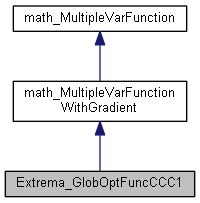Open CASCADE Technology  7.0.0

# Extrema_GlobOptFuncCCC1 Class Reference

This class implements function which calculate Eucluidean distance between point on curve and point on other curve in case of C1 and C2 continuity is C1. More...

`#include <Extrema_GlobOptFuncCC.hxx>`

Inheritance diagram for Extrema_GlobOptFuncCCC1:[legend]

## Public Member Functions

Extrema_GlobOptFuncCCC1 (const Adaptor3d_Curve &C1, const Adaptor3d_Curve &C2)

Extrema_GlobOptFuncCCC1 (const Adaptor2d_Curve2d &C1, const Adaptor2d_Curve2d &C2)

virtual Standard_Integer NbVariables () const
Returns the number of variables of the function. More...

virtual Standard_Boolean Value (const math_Vector &X, Standard_Real &F)
Computes the values of the Functions <F> for the variable <X>. Returns True if the computation was done successfully, False otherwise. More...

virtual Standard_Boolean Gradient (const math_Vector &X, math_Vector &G)
Computes the gradient <G> of the functions for the variable <X>. Returns True if the computation was done successfully, False otherwise. More...

virtual Standard_Boolean Values (const math_Vector &X, Standard_Real &F, math_Vector &G)
computes the value <F> and the gradient <G> of the functions for the variable <X>. Returns True if the computation was done successfully, False otherwise. More...Public Member Functions inherited from math_MultipleVarFunction
virtual Standard_Integer GetStateNumber ()
return the state of the function corresponding to the latestt call of any methods associated to the function. This function is called by each of the algorithms described later which define the function Integer Algorithm::StateNumber(). The algorithm has the responsibility to call this function when it has found a solution (i.e. a root or a minimum) and has to maintain the association between the solution found and this StateNumber. Byu default, this method returns 0 (which means for the algorithm: no state has been saved). It is the responsibility of the programmer to decide if he needs to save the current state of the function and to return an Integer that allows retrieval of the state. More...

virtual ~math_MultipleVarFunction ()

## Detailed Description

This class implements function which calculate Eucluidean distance between point on curve and point on other curve in case of C1 and C2 continuity is C1.

## Constructor & Destructor Documentation

 Extrema_GlobOptFuncCCC1::Extrema_GlobOptFuncCCC1 ( const Adaptor3d_Curve & C1, const Adaptor3d_Curve & C2 )
 Extrema_GlobOptFuncCCC1::Extrema_GlobOptFuncCCC1 ( const Adaptor2d_Curve2d & C1, const Adaptor2d_Curve2d & C2 )

## Member Function Documentation

 virtual Standard_Boolean Extrema_GlobOptFuncCCC1::Gradient ( const math_Vector & X, math_Vector & G )
virtual

Computes the gradient <G> of the functions for the variable <X>. Returns True if the computation was done successfully, False otherwise.

 virtual Standard_Integer Extrema_GlobOptFuncCCC1::NbVariables ( ) const
virtual

Returns the number of variables of the function.

 virtual Standard_Boolean Extrema_GlobOptFuncCCC1::Value ( const math_Vector & X, Standard_Real & F )
virtual

Computes the values of the Functions <F> for the variable <X>. Returns True if the computation was done successfully, False otherwise.